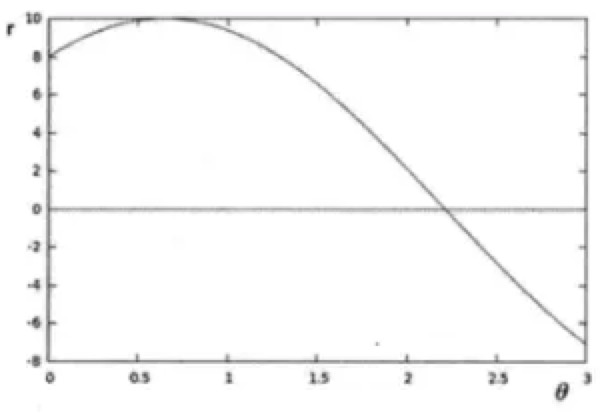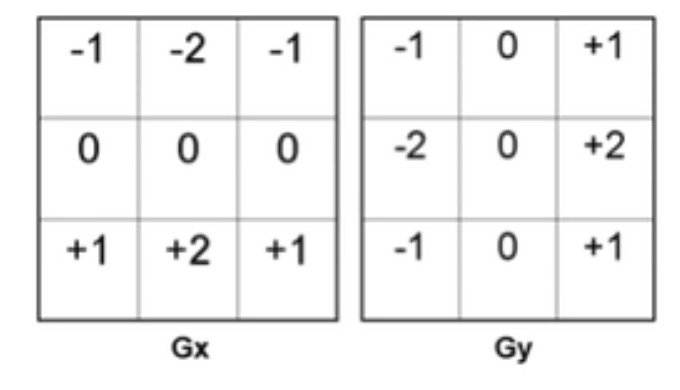# 一些概念

## HSVHSV也叫HSB，俩是同一个东西。还有个HSL，略微有些区别，我也研究，感兴趣参看知乎这篇

• HSB（也就是HSV）中的 H ：好理解，大家都明白，就是啥颜色。
• HSB（也就是HSV）中的 S ：控制纯色中混入$\color{red}{白色}$的量，值越大，白色越少，颜色越纯；
• HSB（也就是HSV）中的 B ：控制纯色中混入$\color{red}{黑色}$的量，值越大，黑色越少，明度越高；噢！对，这个就是HSV的V。

HSV和RGB可以逐个像素转换，转化公式过于亮眼，我也没啥兴趣，就不列出了，感兴趣的自行谷歌吧。

# Hough变换

## 原理• Hough变换通过映射，将一个形状识别问题，转换成了一个统计问题
• 所以，没法做全图的，计算量太大了，一般都是转成二值化的图后，只算为1的点，这样计算量就降下来了
• 找到的正弦的点后，这个点被重叠下很多次，这个点就是很确信是一个直线了，$\color{red}{共线足够多，原图中就存在一条直线}$

## 实践

opencv中有个函数，帮你干这事：

    lines = cv2.HoughLines(
image=edges,
rho=1,
theta=np.pi/180,
threshold=100,
lines=minLineLength,
srn=maxLineGap)


# Canny 边缘检测

## 索贝尔算子Sobel计算梯度• 他实际上是做了一个平滑处理：中间那个-2来说实现的。
• 然后他做了一个梯度处理：[-2,0,2],是的

$Edge Gradient(G)=\sqrt{G_x^2 + G_y^2}$

$Angle(\theta)=tan^{-1}\left( \frac{G_y}{G_x} \right)$

• 梯度的模
• 梯度的角度

## 非极大值抑制(NMS)### 线性差值

$c$点的梯度不是有方向么，就是这条蓝色的线，你看到了吧。他和$g1,g2$所组成的直线，相交在$O$点。 于是，我们就用线性插值，算出$O$到点的梯度值（其实是梯度的模）。这个使用一个三角函数计算，就可以得出。比如$\theta=60$度，也就是$tan(\theta)=\sqrt{3}$，图像中，两个像素距离都是1，那么可以推导出，$|Og2|=1，|g1g2|=|g2C|=\sqrt{3}$，根据这个比例，再加上$g1,g2$上的梯度模差，可以线性插值算出$O$点的梯度模的值。（恩，这玩意是基于$g1,g2$的梯度模值是线性变换的，恩，这个假设很重要！）

## 实践

Canny边缘检测的效果相当不错，实践中推荐多多使用它。

    #image, threshold1, threshold2, edges=None, apertureSize=None, L2gradient=None
edges = cv2.Canny(image=img,
threshold1=10,
threshold2=100,
apertureSize=3,


# SIFT：同一物体映射

SIFT：Scale-invariant feature transform(尺度不变特征变换)

## SIFT干啥用的

SIFT就是想判断两张图片那部分是相同的，

##